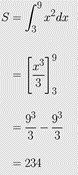## Removing YAML from an RMD file through an R script

So you want to write a book from many RMD files using bookdown.  Here is an R script that strips the YAML lines of an Rmd file because bookdown does not accept files with its own YAML. A new file is created that has no YAML.  The program is written just for one Rmd file, and I will be writing an extension of it to do this for a whole directory including subdirectories.  I will soon write a script that will move the YAML absent RMD files and associated sub-directories to a new directory of its own.

# A program to remove YAML from an RMD file.
# It can be modified to do this for all
# files in a directory and/or its subdirectories
# Stay tuned for the update.

#Set the working directory
setwd("C:/Users/yoda/Rmd")

# filename of the rmd file whose YAML you want to take out
fileName <- "Chapter01.03RoundoffErrors/0103RoundOffErrorsYaml.rmd"

# Open the file to read
Input_File <- file(fileName,open="r")

# icapture is a vector which will check the two lines
# that have --- in them.
icapture <- vector(,10)

# Just printing the lines in the rmd file, not needed.
#for (i in 1:length(linn)){
# print(linn[i])
#}

#The name of the file which will store YAML free RMD file.
YAML_Remove_File <- file("Chapter01.03RoundoffErrors/0103RoundOffErrorsYamlRemove.rmd",open="w")

j <- 0
#Capturing the two line numbers where --- exists
for (i in 1:length(linn)){
if(strtrim(linn[i],3) == "---"){
j <- j+1
icapture[j] <- i
}

# Write to the output file only if it has already captured two ---
if ((j==2) & (i!=icapture)){
writeLines(linn[i],con2)
}
}

#close the input and output files
close(Input_File)
close(YAML_Remove_File)

____________________________

This post is brought to you by

• Holistic Numerical Methods Open Course Ware:
• the textbooks on
• the Massive Open Online Course (MOOCs) available at

## Useful hints for a newbie on Rmarkdown

An R Markdown newbie walks on eggshells so as to not look naïve.  But the community is so nice to them, and since the help is all in the open, it reaches many more.  So here are a few items I learned beyond the usual R Markdown and the ubiquitous example about plotting something about cars.

##### Not Autonumbering

Don’t want to autonumber. If you write (1) First, it will automatically start numbering it. Convenient, but I do not like it sometimes. So how does one stop items from autonumbering. Just put a period sign after (1), that is, (1).  Here is an example.  This will keep the numbering the same as what is inputted.

(1).first
(2).second
(2).second
(3).numbering went away
##### Not wanting to center a block equation

Do you not want a block equation (an equation on its own separate line as opposed to being part of the text which is called an inline equation) to not be in the center, but wanted it tabbed instead.  It is easy to center equations by writing them within two double dollars symbols, that is, .  But I do not like centering.  I like my block equations tabbed.

The equation
$latex a_{11} x_1+a_{12} x_2+\cdots+a_{1n}x_n=b_1$
gets centered by entering as

$$a_{11} x_1+a_{12} x_2+\cdots+a_{1n}x_n=b_1$$

The following, however, put tabs in the equation. Each &emsp puts 4 spaces.  Note also the two dollar symbols, that is, , bounding the equation

&emsp;&emsp;$a_{11} x_1+a_{12} x_2+\cdots+a_{1n}x_n=b_1$
##### Defining often used equations

If you are using certain equation parts, again and again, you can define them.  See  here we are defining $latex \overline{X}$ and $latex \sum_{i=1}^{n}$

{=tex}
\def\Xbar{\overline{X}}
\def\sumn{\sum_{i=1}^{n}}

##### Aligning equations to a character

Many times, you may have equations that are aligned by a character, say an equal to sign.  But if the equations get centered, the equal to sign may not get centered.  This is simply done by adding a & before the aligned character in all lines.  For example, if you want to show the following,you would enter it as the following.  Note where the & is.

\begin{align} \ S &= \int_{3}^{9}{x^2 dx}\\ &= \left[\frac{x^3}{3}\right]_3^9\\ &= \frac{9^3}{3}-\frac{9^3}{3}\\ &= 234 \end{align}

____________________________

References: An Example R Markdown http://statpower.net/Content/311/R%20Stuff/SampleMarkdown.html

This post is brought to you by

• Holistic Numerical Methods Open Course Ware:
• the textbooks on
• the Massive Open Online Course (MOOCs) available at